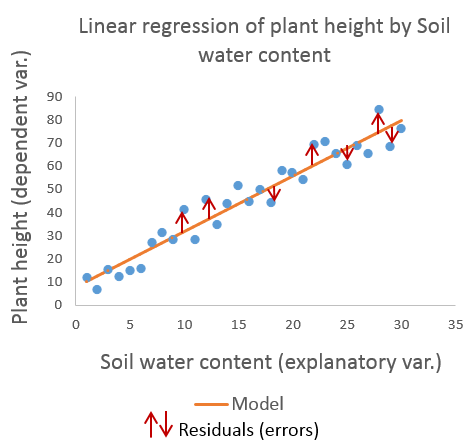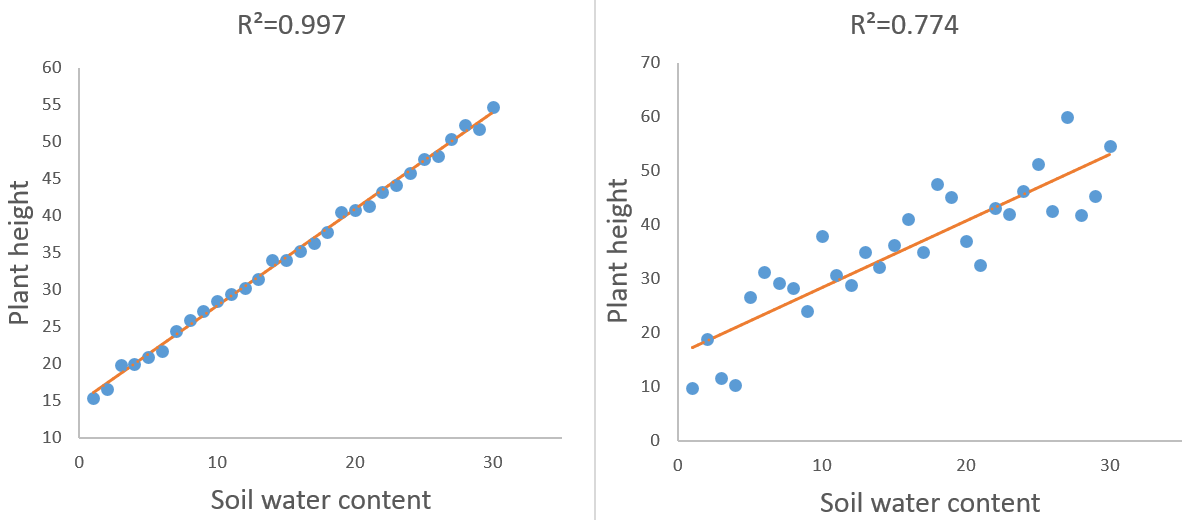Your data analysis solution

# What is statistical modeling?

2017-07-25

## What is statistical modeling?

In simple terms, statistical modeling is a simplified, mathematically-formalized way to approximate reality (i.e. what generates your data) and optionally to make predictions from this approximation. The statistical model is the mathematical equation that is used.

Here is a basic example. Suppose you want to report the weight of a variety of potatoes. We will consider a hard and an easy way to do it. The hard way is spending years measuring the weight of every single potato of this variety in the world, and reporting your data in an endless Excel spreadsheet. The easy way is selecting a 30 potato-wide representative sample of this variety, computing its average and standard deviation and reporting only those two numbers as an approximate description of this weight. Representing a quantity by an average and a standard deviation is a very simple form of statistical modeling.

Another example is attempting to represent the height of plants according to soil water content by a straight line characterized by a slope and an intercept drawn after an experiment on a sample of plants submitted to a growing soil humidity. This particular model is called simple linear regression.## What are dependent and explanatory variables?

In almost all cases, statistical models imply explanatory and dependent variables.

The dependent variable is the one we want to describe, to explain, to predict. As a rule of thumb, the dependent variable is often the one we represent on the Y axis in modeling charts. In the plant height example, the dependent variable is plant height.

Explanatory variables, also referred to as independent variables, are the ones we use to explain, to describe or to predict the dependent variable(s). Explanatory variables are often represented on the X axis. The plant height example involves only one explanatory variable, which is quantitative: soil water content.

Both dependent and explanatory variables may be single or multiple, quantitative or qualitative. There are models adapted to different situations.

## What is a model parameter?

In classic, parametric models, the dependent variable(s) is linked to the explanatory ones through a mathematical equation (the model) that involves quantities called model parameters. In the plant height simple linear regression example, parameters are the intercept and the slope1. The equation may be written like this:

Height = intercept + slope*soil water content

Computations behind statistical modeling allow the estimation of model parameters and further predictions of the dependent variable.

1The simple linear regression also involves a third parameter, the variance of residuals (see paragraph below).

## What is a model residual?

Technically, model residuals (or errors) are the distances between data points and the model (which is represented by the straight line in the plant height linear regression example).Model residuals represent the part of variability in the data the model was unable to capture. The R² statistic is the part of variability that is explained by the model. So the lower the residuals, the higher the R² statistic.## What statistical model should you choose?

This grid will guide you through the choice of the most commonly used models according to the type and number of dependent and independent variables. Solutions other than parametric models are also proposed.

1c26995d494fb3061dd0ae8571ffc0a4@xlstat.desk-mail.com
https://cdn.desk.com/
false
desk